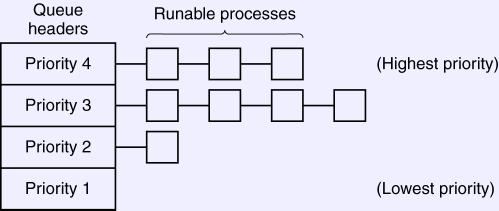Process Scheduling1. Intro.
1. Policy for deciding which process to move to the running state.
2. Traditionally, only one CPU to schedule. Not so much now.
2. Properties.
1. We view jobs as alternatively running on the CPU or waiting for i/o.
2. Periods running the CPU are called bursts. We schedule bursts.
3. Jobs with long bursts are called CPU-bound.
4. Jobs with few or short bursts are called I/O-bound.
5. As CPUs get faster, jobs become more I/O-bound.
3. Batch Scheduling Algorithms.
1. First-Come First-Served (FCFS, also sometimes FIFO).
2. Shortest Job First (SJF).
3. Shortest Remaining Time (SRT). Pre-emptive version of SJF.
4. Last two assume job execution is time is known in advance. For frequently-run batch jobs, this may be true.
4. Interactive Scheduling.
1. Round-Robin Quantum 1, Quantum 4.
2. Priority Scheduling/Multiple Queues.1. Let the highest priority job run.
2. Move down when a full quantum is used.
3. Perhaps move up a job that has been stuck in a low queue a long time.
4. May have longer quanta in lower-priority queues to reduce overhead for CPU-bound jobs.
3. Shortest Process Next.
1. Same as SJF, using a running average of bursts over time as a predictor.
2. Ti is the actual time of the i-th burst.
3. Si is the predicted execution time of the i-th burst, with S0 being a pure guess.
4. Sn+1 = aTn+(1−a)Sn, where 0 ≤ a ≤ 1 is an arbitrary parameter.
5. Larger a favors recent measurements; smaller gives a longer-term average.
6. For a = 1, Sn+1 = Tn: Guess the next burst will be the same as the last.
7. For a = 0.8, Sn+1 = 0.8Tn + 0.16Tn−1 + 0.032Tn−2 + 0.0064Tn−3 + ...
8. For a = 0.5, Sn+1 = 0.5Tn + 0.25Tn−1 + 0.125Tn−2 + 0.03125Tn−3 + ...
9. For a = 0, Sn+1 = S0.
4. Guaranteed Scheduling.
1. Give each process an equal portion of the CPU time.
2. Keep track of CPU time used by each job.
3. For each job, compute a entitled amount of time as the time in system divided by the number of jobs
4. For each job, take the ratio of the actual cpu time over the amount entitled.
5. Run the job with the lowest ratio until it catches up.
5. Lottery scheduling.
1. Give every job some number of tickets.
2. Pick a ticket at random, and run that job.
3. Different numbers of tickets give different priorities.
4. Does not discriminate (for or against) older jobs.